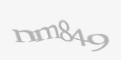Blog

360dissertations understands how tough Masters level projects can be. The standard of a post graduate course is higher than a bachelor’s degree.

Big data refers to dealing with a huge amount of raw data that can be gathered, stored and analyzed to determine the pattern or trend pertaining to the data. As per the data experts by 2020, about 40 trillion gigabytes of data. Although obtaining an analytical picture of data is important, the hard reality is focusing on specific details among the availability of a vast amount of raw data is easier said than done. This is when big data visualization comes into the picture.

Big data visualization is the process of transforming analyzed data into a readable visual format. This is achieved by presenting the data in the form of charts, graphs, tables, and other forms of visuals. With the growing need for presenting the data in readable formats, data visualization tools have gained importance than ever. Some of the popular big data visualization tools that one must look forward to are:

1. Kemal Density estimation for non-parametric Data:- Non-parametric data is nothing but a form of data in which the information pertaining to the population and the underlying distribution of data is unknown. This form of information can be visualized using the Kernel density function. The Kernel density function represents the probability distribution of random variables. This technique is generally used when the parametric distribution of data doesn’t add sense and random data assumptions need to be avoided.

2. Box & Whisker plot for huge data:- A binned box with Whiskers represents the outliers and distribution of the large data. This technique includes five statistics such as minimum, lower quartile, median, upper quartile and maximum to summarize the distribution of data set. The upper quartile will be represented by the upper edge of the box, on the other hand, the lower quartile is described by the lower edge of the box. The minimum & maximum value are represented by Whiskers, and the median is defined by a central line that segregates into sections.

3. Network diagrams & word clouds:- Semistructured and unstructured data require unique visualization techniques. Word cloud technique, often used on unstructured data, demonstrates the frequency (high or low) of a word within the body of text with its size in the cloud. On the other hand, network diagrams can be used on both semistructured and unstructured data. Here, the relationships (individual actors within the network) are represented as nodes and ties (relationships between individuals).

4. Correlation matrices:- This technique combines big data and fast response times and lets you determine the relationships between the variables. The correlation matrix includes a table to display the correlation coefficient between variables. Each cell will demonstrate t relationship between the two variables. A correlation matrix can also be used to summarize the data, as a diagnostic for advanced analysis and as an input to further advanced analysis.

5. Line charts:- Also known as the row graph, line charts are the simplest form of data visualization technique that can be used for big data as well as traditional data. This type of chart includes a graph of information represented using a number of rows. The line chart plots the dependency or relationship of one variable on another.

6. Heat maps:- A heat map is a form of data visualization technique which represents the displayed information in two dimensions by color values. This technique provides an instant overview of the data pattern. Heat maps or thermal maps can be of various types. However, note that all the maps will transmit interactions between information values and use different colors that are difficult to comprehend.

7. Histogram plot:- A histogram plot is one of the commonly used data visualization techniques in the big data field. This technique represents the distribution of continuous variables over a given period or interval of time. Histogram plots the data by dividing it into small intervals known as bins. This plot can also be used to investigate the underlying distribution, skewness, frequency, outliers, and many more.

To conclude, consider the requirements of your study prior to choosing the data visualization technique. This is because if the data pattern is not identified and isn’t represented accurately, then the data collected will not add value to your study.